﻿ Capacitance Change by Thermal ExpansionExamples | Product | Murata Software Co., Ltd.# Example2Capacitance Change by Thermal Expansion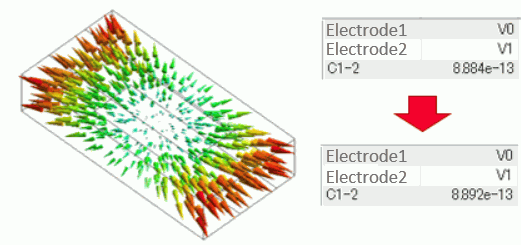General

• Capacitance change of a capacitor by thermal expansion is analyzed.

• [Analysis with deformed shape taken into consideration] is used.

• Unless specified in the list below, the default conditions will be applied.

Three analysis models are prepared.

・Before deformation (electric field analysis)

・Deformed shape (mechanical stress analysis)

・After deformation (electric field analysis)

See [Analysis with the Deformed Shape Taken into Account] for more information.

### Analysis Model 1: Before Deformation

Set up analysis before the deformation. The model is named [Before deformation].

### Analysis Space

 Item Setting Analysis Space 3D Model unit mm

### Analysis Condition

 Item Setting Solver Electric field analysis [Coulomb] Analysis Type Static analysis (capacitance) Options None

In actuality, the electric field exists outside the analysis domain. Therefore the open boundary condition below is applied initially.

 Tab Setting Item Setting Open Boundary Tab Type Absorbing boundary Order of Absorbing Boundary 1st degree

### Graphical Object

Create a cubic solid body for a dielectric (Block).

Create the voltage-setting places by the sheet bodies to set the voltage boundary condition on the top and bottom faces of the dielectric.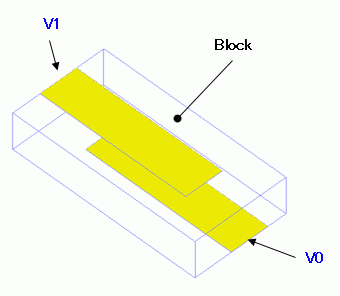### Body Attributes and Materials

 Body Number/Type Body Attribute Name Material Name 0/Solid Block 001_Alumina * 1/Sheet None (imprinting body) 2/Sheet None (imprinting body)

* Available from the material DB

### Boundary Condition

 Boundary Condition Name/Topology Tab Boundary Condition Type Setting V0/Face Electric Electric wall Voltage specified, 1[V] V1/Face Electric Electric wall Voltage specified, 0[V] Outer Boundary Condition * Electric Open Boundary Set up on the open boundary tab.

To set Outer Boundary Condition, go to the [Model] taband click [Outer Boundary Condition]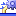.

### Analysis Model 2: Deformed Shape Calculation

Set the mechanical stress analysis to calculate the deformation caused by the thermal expansion.

Copy Analysis Model 1 (before deformation) and set up as follows. The model is named [Deformed shape calculation].

See [Copy Analysis Model into Project] for the details.

### Analysis Condition

 Item Setting Solver Mechanical stress analysis [Galileo] Analysis Type Static Analysis Options Thermal Load

 Tab Setting Item Setting Step/Thermal Load Step Setting Thermal load analysis Reference temperature 25[deg] Reached Temperature 200[deg]

### Analysis Model 3: After Deformation

Set up the analysis that takes the deformed shape into account.

Copy Analysis Model 1 (before deformation) and set up as follows. The model is named [After deformation].

See [Copy Analysis Model into Project] for the details.

### Analysis Condition

Specify Analysis Model 2 (Deformation shape calculation) on the result import tab.

 Tab Setting Item Setting Result Import Import Type Deformed shape Specify Results Specify Analysis Model [Deformed Shape Calculation]

### Run Mesher/Solver

Run the solver with all analysis models. Calculation of Analysis Model 2 (deformed shape) must be completed before going on to Analysis Model 3 (after deformation).

### Results

To see the calculation results, go to the [Results] taband click [Table]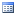.

Results of Analysis Model 1 (before deformation) are as follows.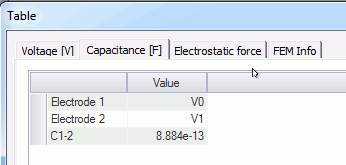The capacitance of C1-2 is 0.884[pF].

A displacement diagram of Analysis Model 2 (deformed shape calculation) is shown as below.

The expansion due to the temperature change is observed.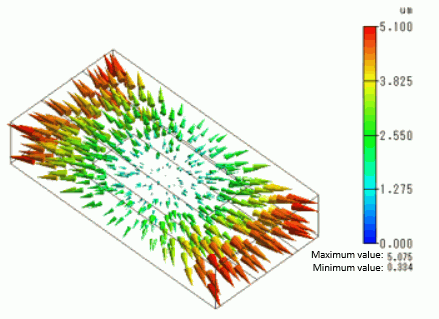Results of Analysis Model 3 (After deformation) are as follows.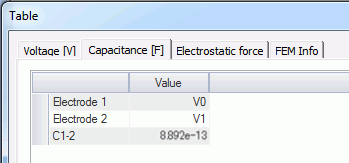The capacitance of C1-2 is 0.892[pF]. It confirms that the capacitance is increased due to the deformation.

﻿